# 13.1 BinaryTrie: A digital search tree

A BinaryTrie encodes a set of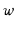bit integers in a binary tree. All leaves in the tree have depthand each integer is encoded as a root-to-leaf path. The path for the integer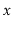turns left at level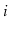if theth most significant bit ofis a 0 and turns right if it is a 1. Figure 13.1 shows an example for the case, in which the trie stores the integers 3(0011), 9(1001), 12(1100), and 13(1101).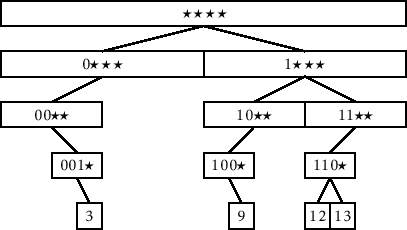Because the search path for a valuedepends on the bits of, it will be helpful to name the children of a node,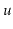,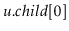(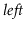) and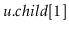(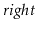). These child pointers will actually serve double-duty. Since the leaves in a binary trie have no children, the pointers are used to string the leaves together into a doubly-linked list. For a leaf in the binary trie(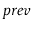) is the node that comes beforein the list and(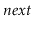) is the node that followsin the list. A special node,, is used both before the first node and after the last node in the list (see Section 3.2).

Each node,, also contains an additional pointer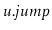. If's left child is missing, thenpoints to the smallest leaf in's subtree. If's right child is missing, thenpoints to the largest leaf in's subtree. An example of a BinaryTrie, showing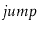pointers and the doubly-linked list at the leaves, is shown in Figure 13.2.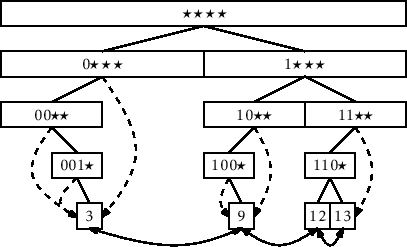The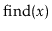operation in a BinaryTrie is fairly straightforward. We try to follow the search path forin the trie. If we reach a leaf, then we have found. If we reach a nodewhere we cannot proceed (becauseis missing a child), then we follow, which takes us either to the smallest leaf larger thanor the largest leaf smaller than. Which of these two cases occurs depends on whetheris missing its left or right child, respectively. In the former case (is missing its left child), we have found the node we want. In the latter case (is missing its right child), we can use the linked list to reach the node we want. Each of these cases is illustrated in Figure 13.3.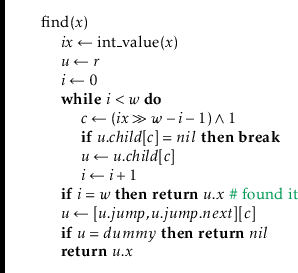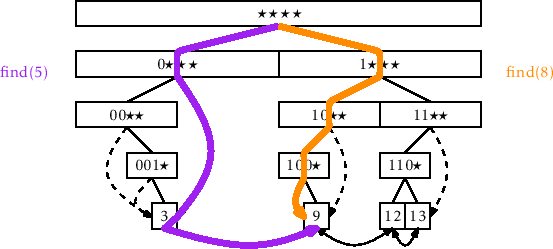The running-time of themethod is dominated by the time it takes to follow a root-to-leaf path, so it runs in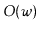time.

The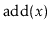operation in a BinaryTrie is also fairly straightforward, but has a lot of work to do:

1. It follows the search path foruntil reaching a nodewhere it can no longer proceed.
2. It creates the remainder of the search path fromto a leaf that contains.
3. It adds the node,, containingto the linked list of leaves (it has access to the predecessor,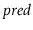, of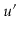in the linked list from thepointer of the last node,, encountered during step 1.)
4. It walks back up the search path foradjustingpointers at the nodes whosepointer should now point to.
An addition is illustrated in Figure 13.4.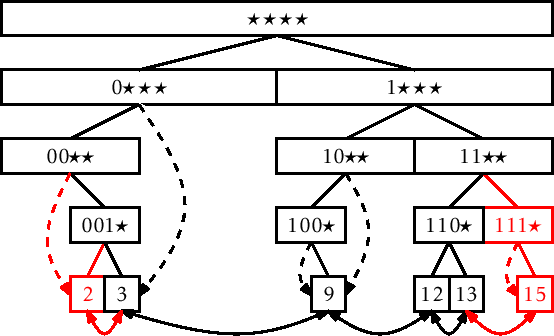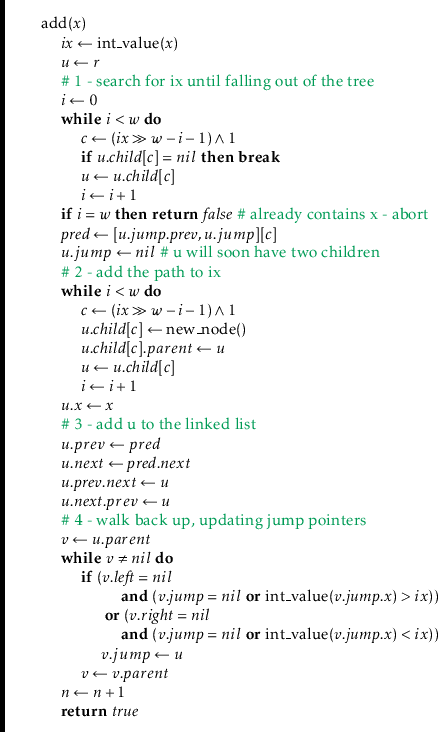This method performs one walk down the search path forand one walk back up. Each step of these walks takes constant time, so themethod runs intime.

The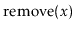operation undoes the work of. Like, it has a lot of work to do:

1. It follows the search path foruntil reaching the leaf,, containing.
2. It removesfrom the doubly-linked list.
3. It deletesand then walks back up the search path fordeleting nodes until reaching a node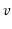that has a child that is not on the search path for.
4. It walks upwards fromto the root updating anypointers that point to.
A removal is illustrated in Figure 13.5.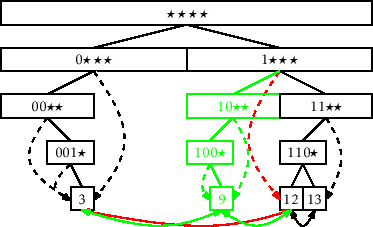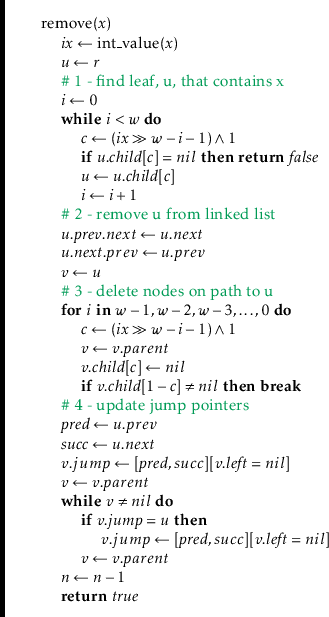Theorem 13..1   A BinaryTrie implements the SSet interface for-bit integers. A BinaryTrie supports the operations,, andintime per operation. The space used by a BinaryTrie that storesvalues is.

opendatastructures.org